Friday , August 23 2019

# Arrays in C Programming

In this tutorial, we’ll learn about arrays in C Programming. Now the question is what are arrays and how are they useful? In all of our previous lessons we’ve used only few variables in each program. What if we need 100 variables of same data type? In this case we need to declare an array; arrays are group of item of same type under one variable name. It is an ordered list of elements where each is of the same data type. A one-dimensional array is represented by specifying the array name followed by a number in square brackets [ ] to indicate the array size or number of elements. Now let’s look at example code.

#### One Dimensional Array

Let’s declare a one dimensional array named myArray. This array contains four integer numbers. Now each block contains one integer number. In this example we have to access this value using address.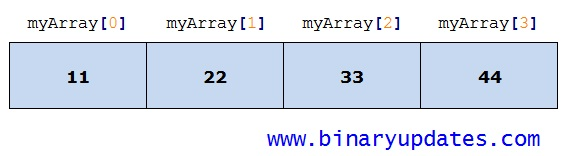One Dimensional Array
##### Example: 1D Arrays in C Programming
Explanation: int myArray this statement initializes an array i.e. myArray. This array is of type integer. The value between [] bracket represents the size of an array. In this case myArray holds four integer numbers. The array organized as a block of memory. Each element in array stored in individual block as represented in picture. And each block has an address depending on your machine. The printf statements prints the value stored and address of each element.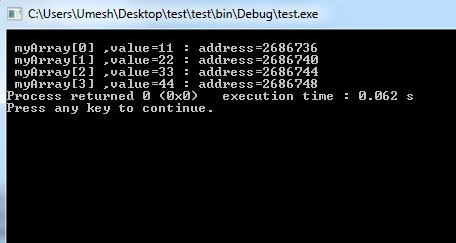1D Array in C Programming

This reveals that arrays are not only stored group of variables but also allows us to manipulate them. This make array as a powerful programming tool. Array shares very special relationship with pointer.

#### Array and Pointer Relationship

Array and pointer share very special relationship with each other. A pointer variable is used to store the address of another variable while an array is used to group related data items of same data type.

The name of the array is a pointer to the first element of the array. That means it holds the address of the very first element of the array. The example below will help you understand this relation.

##### Example: Array and Pointer Relationship
Let’s build and run this example code and observe output. The output from this program is: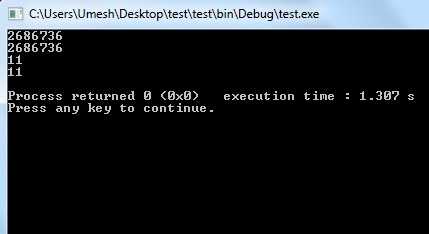Array and Pointer Relationship

Explanation: As per first printf statement, printing A will print the address of first element. Also printing &A prints same address 2686736. This means when we print array usually it prints starting address of array. This is same as address of A. When we print A, we will get the value of first element in array i.e. 11. As Array indexing starts with 0. Now when we print *A we will get same value 11 printed on screen. This means when we use pointer to an array name. This pointer points to starting address of an array.

As we know from our previous post pointers in C. We can use pointer arithmetic and loops to enhance use of Arrays in C Programming. We will dive deep in future lesson.

#### Multi-dimensional Arrays in C

The most basic form of multi-dimensional array is two dimensional arrays. The process of initialization of two dimensional arrays is same as one dimensional array. The only difference is we need two subscripts to declare two dimensional array. For example: int A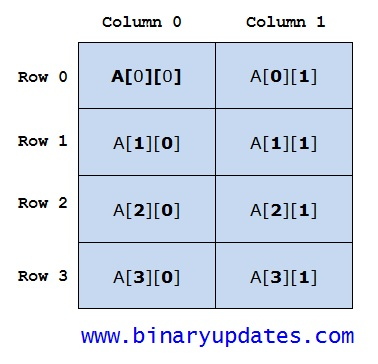Two Dimensional Arrays

Two dimensional (2D) array are generally known as matrix. The image above is an memory representation of 2D array A. In this example ‘A’ is a name of an array, 4 and 2 are subscripts. Where 4 rows and 2 column uniquely identify each element in ‘A’. The example code below shows you how to declare 2D array. We will going to access individual element of an array.

Let’s build and run this example code and observe output. The output from this program is: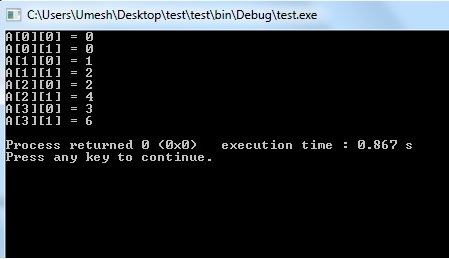Output of Two-dimensional Arrays

As we have seen in this example. We can create arrays of any dimension. The most commonly used arrays are one or two dimensional. You may need further dimensional arrays in your program. This all depends on requirement and application itself.

#### Array as Function Argument in C

We can pass single dimensional array as an argument in a function. To do this we would have to declare formal parameter in one of following three ways and all three declaration methods produce similar results because each tells the compiler that an integer pointer is going to be received.

1st Way

2nd Way

3rd Way

##### Example: Array as a Function Argument

Let’s take a look at example code. We have to pass an array ‘A’ to a function SumOfElements. It returns the sum of the numbers passed through the array, A[] = {1, 2, 3, 4, 5}.

Now let’s run this code and We will get output shown below: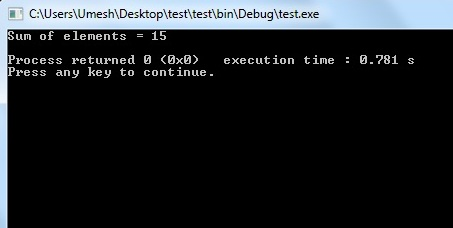Output of Array as Function Argument

#### Pointer as a Function Argument

##### Example: Pointer as a Function
This is it for Arrays in C Programming. In next lesson we’ll learn about String in C Programming. Please do write us if you have any suggestion/comment or come across any error on this page. Thanks for reading.

### About Umesh LokhandeUmesh Lokhande holds a Master degree in Scientific Instrumentation from University of Applied Sciences Jena, Germany. and has previously worked at Orbotech, Alere Technologies etc. Umesh is also a founder and first author of BINARYUPDATES.COM